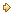Visitors Online: 77 | Sunday 20th October 2019

CBSE Guess > Papers > Question Papers > Class XII > 2004 > Economics > Compartment Delhi Set-II

ECONOMICS (Set II—Compartment Delhi)

Except for the following questions, all the remaining questions have been asked in Set I.

SECTION - A

Q.1. Answer the following questions 1x4
(i) Give one example of variable cost.
(ii) Give meaning of producer’s equilibrium.
(iii) Define production function.
(iv) Give meaning of opportunity cost.

Q. 4. What is the relation between the change in the price of a good and the change in demand of its complementary good? Explain with the help of an example. 1, 2

Q. 6. Explain ‘product homogeneity’ feature of perfect competition. 4
Or
Explain ‘free entry and exit of firms’ feature of perfect competition.

Q. 8. Given that Total Fixed Cost is Rs. 30, calculate (a) Marginal Cost and (b) Total Cost from the following: 4

 Output (Units) Total variable Cost (Rs.) 0 1 2 3 0 20 50 100

Q. 9. At a price of Rs. 6 per unit a consumer buy 50 units of a good. The price elasticity of demand as -2 At what price will the consumer buy 100 units? 4

SECTION – B

Q. 13. Answer the following questions: 1x4
(i) What is Balance of Trade?
(ii) Define foreign exchange.
(iii) Give one e of micro economics
(iv) Give meaning of macro economics

Q. 14. Explain the relation between foreign exchange rate and supply of foreign exchange. 3

Q. 16. If marginal propensity to consume is 0.8 and total increase in national income is Rs. 500 crores, find out increase in investment. 3

Q. 17. Calculate Gross Value Added at Market Price from the following: 3

 Rs. (Lakhs) (i) Intermeitae consumption (ii) Closing stock (iii) Purchase of materials (iv) Excise tax (v) Sales (vi) Depreciation (vii) Opening stock 60 2040 10 300 30 20

Q. 22. Find out (a) National Income and (b) Gross National Disposable Income from the following data: 4, 2

 Rs. (Crores) (i) Imports (ii) Government final consumption expenditure (iii) Net factor income from abroad (iv) Net current transfers from abroad (v) Private final consumption expenditure (vi) Subsidies (vii) Indirect tax (viii) Net domestic capital formation (ix) Exports: (x) Consumption of fixed capital (depreciation) 20 60 (-) 5 5 200 10 40 70 2015

 Economics 2004 Question Papers Class XII Delhi Outside Delhi Compartment Delhi Compartment Outside DelhiSet ISet ISet ISet ISet IISet IISet IISet IIISet III

CBSE 2004 Question Papers Class XII